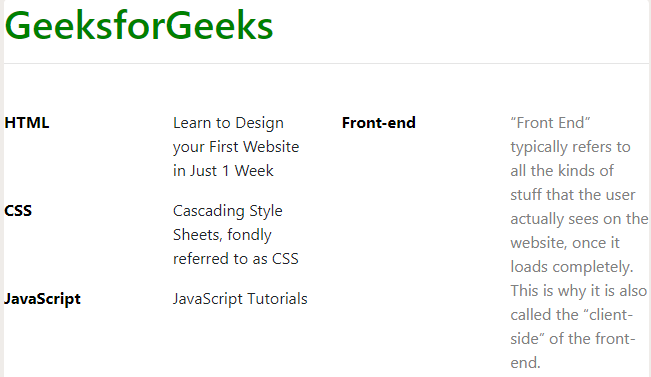# How to use Bootstrap to align labels with content into 4 columns ?

• Last Updated : 08 Jul, 2019

The motive of this article is to align the content into four columns where the first two columns denote the labels and its content and the last two columns denote the labels and its content. The class “row” and “col” are used to create a grid which can be represented by a number of rows and columns. The class “row” creates a row into which the content will be placed and the class “col” creates partitions in that row making space for 4 labels or 4 items to be placed in that row.
This example uses Bootstrap to create four columns where the first two columns represent the labels and its content and the last two columns represent the labels and its content.
Example:

 ```<``html``>`` ` `<``head``>``    ``<``title``>``        ``How to use Bootstrap to align labels``        ``with content into 4 columns ?``    ````     ` `    ``<``link` `rel``=``"stylesheet"` `href``=``"https://stackpath.bootstrapcdn.com/bootstrap/4.3.1/css/bootstrap.min.css"``>``    ``<``link` `rel``=``"stylesheet"` `type``=``"text/css"` `href``=``"https://stackpath.bootstrapcdn.com/font-awesome/4.7.0/css/font-awesome.min.css"``>`` ` `    ``<``style``>``        ``form {``            ``margin-top: 30px;``            ``margin-bottom: 30px;``            ``padding-bottom: 25px;``        ``}``        ``.form-area {``            ``margin-top: 30px;``        ``}``        ``label {``            ``display: block;``            ``color: black;``            ``font-weight: bold;``        ``}``    `````` ` `<``body``>``    ``<``h1` `style``=``"color:green"``>GeeksforGeeks``    ``<``hr``>``    ``<``form``>``        ``<``div` `class``=``"row"``>``            ``<``div` `class``=``"col"``>`` ` `                ``<``div` `class``=``"row"``>``                    ``<``div` `class``=``"col"``>``                        ``<``label``>HTML``                    ````                    ``<``div` `class``=``"col"``>``                        ``<``p``>``                            ``Learn to Design your First``                            ``Website in Just 1 Week``                        ````                    ````                ```` ` `                ``<``div` `class``=``"row"``>``                    ``<``div` `class``=``"col"``>``                        ``<``label``>CSS``                    ````                    ``<``div` `class``=``"col"``>``                        ``<``p``>``                            ``Cascading Style Sheets, fondly``                            ``referred to as CSS``                        ````                    ````                ```` ` `                ``<``div` `class``=``"row"``>``                    ``<``div` `class``=``"col"``>``                        ``<``label``>JavaScript``                    ````                    ``<``div` `class``=``"col"``>``                        ``<``p``>JavaScript Tutorials``                    ````                ```` ` `            ```` ` `            ``<``div` `class``=``"col"``>``                ``<``div` `class``=``"row"``>``                    ``<``div` `class``=``"col"``>``                        ``<``label``>Front-end``                    ````                    ``<``div` `class``=``"col"``>``                        ``<``p` `style``=``"font-weight: italic; color: grey"``>``                            ``“Front End” typically refers to all the ``                            ``kinds of stuff that the user actually sees ``                            ``on the website, once it loads completely. ``                            ``This is why it is also called the “client-side”``                            ``of the front-end.``                        ````                    ````                ````            ````        ````    `````` ` `                    `

Output:My Personal Notes arrow_drop_up